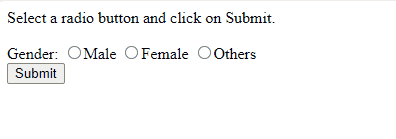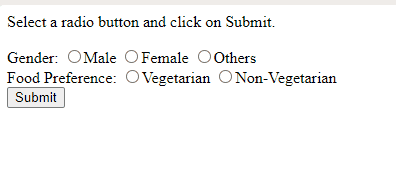Open In App

# How to get value of selected radio button using JavaScript ?

To get the value of the selected radio button, a user-defined function can be created that gets all the radio buttons with the name attribute and finds the radio button selected using the checked property. The checked property returns True if the radio button is selected and False otherwise. If there are multiple Radio buttons on a webpage, first, all the input tags are fetched and then the values of all the tags that have typed as ‘radio’ and are selected are displayed.

Example 1: The following program displays the value of the selected radio button when the user clicks on Submit button.

## html

 ```<``html` `lang``=``"en"``>``<``head``>``    ``<``title``>``          ``How to get value of selected radio button using JavaScript ?``      ````` `<``body``>``    ``<``p``>``        ``Select a radio button and click on Submit.``    ````    ``Gender:``    ``<``input` `type``=``"radio"` `name``=``"gender"` `value``=``"Male"``>Male``    ``<``input` `type``=``"radio"` `name``=``"gender"` `value``=``"Female"``>Female``    ``<``input` `type``=``"radio"` `name``=``"gender"` `value``=``"Others"``>Others``    ``<``br``>``    ``<``button` `type``=``"button"` `onclick``=``"displayRadioValue()"``>``        ``Submit``    ````    ``<``br``>``    ``<``div` `id``=``"result"``>``    ``<``script``>``        ``function displayRadioValue() {``            ``var ele = document.getElementsByName('gender');` `            ``for (i = 0; i < ``ele.length``; i++) {``                ``if (ele[i].checked)``                    ``document.getElementById("result")``.innerHTML``                        ``= ``"Gender: "` `+ ele[i].value;``            ``}``        ``}``    ```````

Output :How to get the value of the selected radio button using JavaScript?

Example 2: The following program displays the values of all the selected radio buttons when submit is clicked.

## html

 ```<``html` `lang``=``"en"``>``<``head``>``    ``<``title``>``          ``How to get value of selected radio button using JavaScript ?``      ````` `<``body``>``    ``<``p``>``        ``Select a radio button and click on Submit.``    ````    ``Gender:``    ``<``input` `type``=``"radio"` `name``=``"Gender"` `value``=``"Male"``>Male``    ``<``input` `type``=``"radio"` `name``=``"Gender"` `value``=``"Female"``>Female``    ``<``input` `type``=``"radio"` `name``=``"Gender"` `value``=``"Others"``>Others``    ``<``br``>``    ``Food Preference:``    ``<``input` `type``=``"radio"` `name``=``"Food"` `value``=``"Vegetarian"``>Vegetarian``    ``<``input` `type``=``"radio"` `name``=``"Food"` `value``=``"Non-Vegetarian"``>Non-Vegetarian``    ``<``br``>``    ``<``button` `type``=``"button"` `onclick``=``"displayRadioValue()"``>``        ``Submit``    ````    ``<``br``>``    ``<``div` `id``=``"result"``>``    ``<``script``>``        ``function displayRadioValue() {``            ``document.getElementById("result").innerHTML = "";``            ``var ele = document.getElementsByTagName('input');``            ``for (i = 0; i < ``ele.length``; i++) {``                ``if (ele[i]``.type` `= ``"radio"``) {` `                    ``if (ele[i].checked)``                        ``document.getElementById("result").innerHTML``                            ``+= ele[i].name + " Value: "``                            ``+ ele[i].value + "
";``                ``}``            ``}``        ``}``    ```````

Output:How to get the value of the selected radio button using JavaScript?

JavaScript is best known for web page development but is also used in various non-browser environments. You can learn JavaScript from the ground up by following this JavaScript Tutorial and JavaScript Examples.